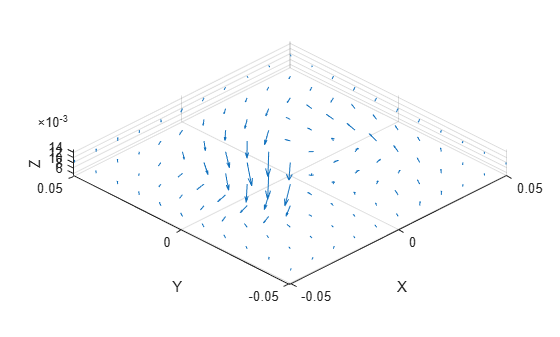# fieldsCustom

Plot electric or magnetic field of antenna

## Syntax

``fieldsCustom(field,points)``
``fieldsCustom(field,points,scalefield)``
``qobj = fieldsCustom(___)``
``fieldsCustom(axeshandle,___)``

## Description

example

````fieldsCustom(field,points)` plots electric or magnetic field vectors, `fields`, at specified points in space, `points`, in the current axes. ```

example

````fieldsCustom(field,points,scalefield)` scales the field arrows by a scalar value, `scalefield`.```
````qobj = fieldsCustom(___)` returns the quiver object, using either of the previous syntaxes.```
````fieldsCustom(axeshandle,___)` plots into the axes specified by `axeshandle` instead of the current axes.```

## Examples

collapse all

Load and visualize the magnetic field data available in the file '`fielddata.mat'`.

```load fielddata fieldsCustom(H,p)```Scale the magnetic field arrows by a factor of 2.

```figure fieldsCustom(H,p,2)```## Input Arguments

collapse all

Complex electric field strength vector in V/m or magnetic field strength vector in A/m at field points, specified as a 3-by-p complex matrix. p is the number of points in space.

Data Types: `double`
Complex Number Support: Yes

Cartesian coordinates x, y, and z of field points, specified as a 3-by-p real matrix. p is the number of points in space.

Data Types: `double`

Axis handle, specified as an axes object.

Data Types: `char`

Value by which to scale the field arrows, specified as a scalar. A value of `2` doubles the relative length of the field arrows. A value of `0.5` halves the length of the field arrows. A value of `0` plots the field arrows without automatic scaling.

Example: `2`

Data Types: `double`

## Output Arguments

collapse all

Electric or magnetic field plot, returned as quiver object.

## Version History

Introduced in R2016a